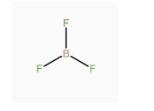QuestionAnswers

# Give reasons:A.$B{F_3}$ has zero dipole moment although the B – F bonds are polar.B.NaCl is harder than Na metal.

A.$B{F_3}$ has zero dipole moment although the B – F bonds are polar.
-Dipole moment in any system is due to the separation of charge. It can arise in ionic bonds as well as covalent bonds. It generally occurs due to the difference in electronegativity between the two chemically bonded atoms. Due to the difference in electronegativity, partial positive charge is induced on one atom and partial negative charge is induced on the other atom. The direction of the dipole moment is from the positive charge to the negative charge. -In the given molecule $B{F_3}$, B – F bonds are polar but the molecule has zero dipole moment because the net dipole moment is the vector sum of all the constituent dipole moments. So, the overall dipole moment will be the vector sum of all the three B – F dipole moments, which are at 120°. The vector sum of any three equal vectors at 120° to each other is equal to zero. Hence, the net dipole moment is zero.